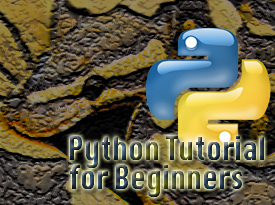# How to print in the same line using end=’ ‘ argumentThe print statement prints by default a newline character in Python. Look this example:

print("Hello")
print("world")

The output is going to be in two separated lines:

Hello
world

If you want to avoid this and use multiple print statements but print in the same line, you have to explicitly tell Python to end its print statement with an empty string instead of a newline character. To do this, you have to add a second argument end=’ ‘ which tells Python to end the print statement with an empty string.

If you are using Python 2.x you are most likely end up with a SyntaxError like this one:This error occurs because print statement is not implemented as a function.

So, if you are using Python 2.x, you need to fix this error by telling Python to use a print statement as a function. To do this, add this line of code before anything else:

from __future__ import print_function

Now, your file looks like this:

from __future__ import print_function
print("Hello", end=' ')
print("world")

If you’re using Python 3 then you don’t have to use from __future__ import print_function because print() is implemented as a function already.

print("Hello", end=' ')
print("world")

and the output will be in the same line

Hello world